# Life Sciences - (XL) 2018 GATE Paper (Practice Test - 4)

## 20 Questions MCQ Test GATE Past Year Papers for Practice (All Branches) | Life Sciences - (XL) 2018 GATE Paper (Practice Test - 4)

Description
Attempt Life Sciences - (XL) 2018 GATE Paper (Practice Test - 4) | 20 questions in 30 minutes | Mock test for GATE preparation | Free important questions MCQ to study GATE Past Year Papers for Practice (All Branches) for GATE Exam | Download free PDF with solutions
QUESTION: 1

Solution:
QUESTION: 2

Solution:
QUESTION: 3

### The oral polio vaccine (OPV) consists of

Solution:
QUESTION: 4

Which of the following eukaryotic cellular components carries out intracellular degradation during autophagy?

Solution:
QUESTION: 5

Analysis of DNA sequences suggest that eukaryotic mitochondrial genomes primarily originated from

Solution:
QUESTION: 6

Binomial nomenclature has NOT yet been adopted for

Solution:
QUESTION: 7

Which of the following is NOT an accepted method for sterilization?

Solution:
QUESTION: 8

The primary product of nitrogen fixation is

Solution:
QUESTION: 9

In humans, the key stages in the life cycle of malarial parasites occur in

Solution:
QUESTION: 10

You have a 50 mg/mL stock solution of arginine. To prepare 1 liter of growth medium for an arginine auxotroph that requires 70 µg/mL of arginine, the volume of this stock solution that should be added is _____________ mL (up to 1 decimal point) .

Solution:
QUESTION: 11

Accumulating evidence suggest that Domain Archaea is more closely related to Domain Eukarya than to Domain Bacteria. Which of the following properties are shared between eukaryotes and archaea ?

(i) Protein biogenesis
(ii) Presence of sterol containing membranes
(iii) Ribosomal subunit structures
(iv) Adaptation to extreme environmental conditions
(v) Fatty acids with ester linkages in the cell membrane

Solution:
QUESTION: 12

Match the antimicrobial agents in group I with their category/mode of action in group II.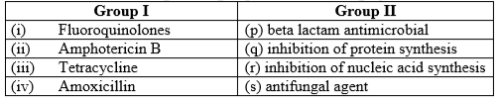Solution:
QUESTION: 13

Match the microorganisms to their predominant modes of transmission.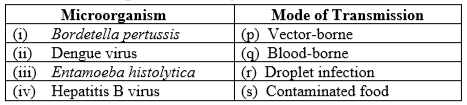Solution:
QUESTION: 14

Match the precursors/intermediates with the corresponding metabolic pathways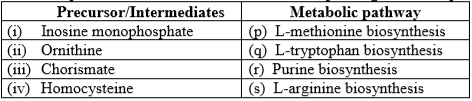Solution:
QUESTION: 15

Match the scientists to their area of major contribution Scientists Area of major contribution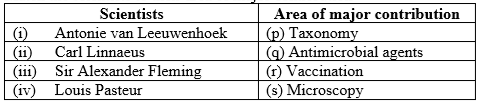Solution:
QUESTION: 16

Which of the following combinations would improve the resolution of a microscope?

(i) Increasing the half aperture angle of the objective lens
(ii) Decreasing the wavelength of the illumination source
(iii) Decreasing the numerical aperture of the objective lens
(iv) Decreasing the refractive index of immersion medium

Solution:
QUESTION: 17

Active transport involves the movement of a biomolecule against a concentration gradient across the cell membrane using metabolic energy. If the extracellular concentration of a biomolecule is 0.005M and its intracellular concentration is 0.5M, the least amount of energy that the cell would need to spend to transport this biomolecule from the outside to the inside of the cell is ________ kcal/mol (up to 2 decimal points).

(Temperature T = 298K and universal gas constant R= 1.98 cal/mol.K)

Solution:
QUESTION: 18

A continuous cell culture being carried out in a stirred tank reactor is described in terms of its cell mass concentration X and substrate concentration S. The concentration of the substrate in the sterile feed stream is SF = 10 g/L and yield coefficient Yx/s = 0.5. The flow rates of the feed stream and the exit stream are equal (F=5 mL/min) and constant. If the specific growth rate  (h-1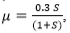the steady state concentration of S is _____ g/L (up to 1 decimal point).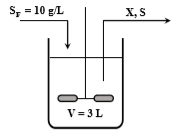Solution:
QUESTION: 19

The initial concentration of cells (N0) growing unrestricted in a culture is 1.0×106 cells/mL. If the specific growth rate (µ) of the cells is 0.1 h-1, the time required for the cell concentration to become 1.0×108 cells/mL is _________ hours (up to 2 decimal points).

Solution:
QUESTION: 20

The following stoichiometric equation represents the conversion of glucose to lactic acid in a cell: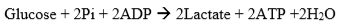If the free energy of conversion of glucose to lactic acid only is ∆G0 = 47000 cal/mol, the efficiency of energy transfer is _______ % (up to 1 decimal point). (∆G0 for ATP hydrolysis is -7.3 kcal/mol)

Solution:Use Code STAYHOME200 and get INR 200 additional OFF Use Coupon Code